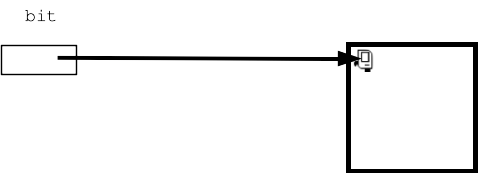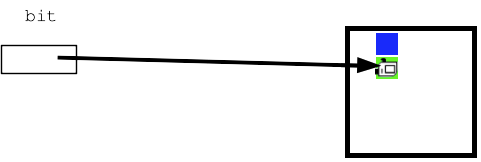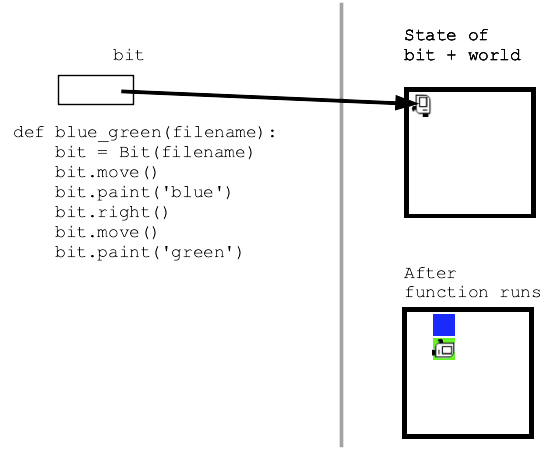# Bit Reference

## Bit Reference

We'll use the simple "bit" robot for our first examples as an on-ramp for Python functions in CS106A. Bit lets us play with 100% standard Python code, but in a way where the results of each line are visual and immediate, so it's a handy way to see how the pieces of code fit together.

The examples showing how to use bit are in the lecture notes; this page is just the reference of bit functions.

The simple bit robot exists in a rectangular world made of little squares. Each square in bit's world can be painted one of 'red' 'green' or 'blue' or left unpainted white. The black squares are solid, and bit can't move there.

The bit robot can take just a few actions in the world - each action corresponds to a Python "function" that performs that action.

• Change bit's facing direction:
`bit.left()`
`bit.right()`
• Move bit forward one square:
`bit.move()`
Bit can only move into empty "clear" squares
The edge of the world does not count as clear
Black filled-in squares are also not clear
Moving to a non-clear square is an error
• Paint the current square:
`bit.paint('red')`
`bit.paint('green')`
`bit.paint('blue')`
An example of passing a parameter into a function when it is called (e.g 'red')
• Test if the way forward is clear before a move:
`bit.front_clear()`
-returns True or False (use with 'while' or 'if')
-True means the square in front is clear and a move would succeed
-False means the square is blocked and a move would be an error
• Test color of current square
`bit.get_color()`
Returns the color of the square bit is on: 'red' 'green' 'blue' or `None` if the square is not colored
`None` is a special Python value for nothing
• Test if the squares to the left or right are clear
`bit.left_clear()`
`bit.right_clear()`
Like front_clear() but to the side
• Erase the current square, removing any painting (new, not used much yet)
`bit.erase()`

Here is a picture of bit at the upper left of a world, before taking some actions:If bit then does these actions:

``````bit.move()
bit.paint('blue')
bit.right()
bit.move()
bit.paint('green')
``````

Then the world will look like this:## Bit Functions

As a practical matter, bit code is inside functions like this:## Bit From Filename

Your code is shielded from the details, but a bit world is stored in a plain text file with a name like '5x5.world' or 'super-spiral.world'.

The function "Bit" (uppercase 'B') is a function which takes a filename parameter (e.g. '5x5.world'), reads the rectangular world into memory with a bit in it, and returns a reference to the bit. A bit always exists within the context of a specific world in this way.

So your code can create a bit in, say, a world named '5x5.world' like this:

``````      bit = Bit('5x5.world')
``````

More often the first line in a function looks like this:

``````def do_something(filename):
bit = Bit(filename)
bit.move()
...
``````

The filename is passed in as a parameter, e.g. '5x5.world', and the first line creates bit from the that filename and then goes ahead.